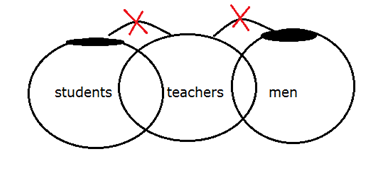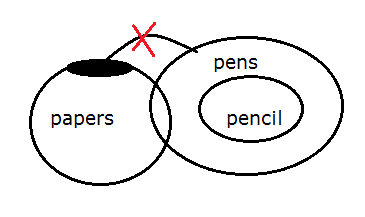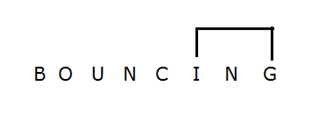# SBI Clerk Pre Reasoning (Day-28)

Dear Aspirants, Our IBPS Guide team is providing new series of Reasoning Questions for SBI Clerk Prelims 2020 so the aspirants can practice it on a daily basis. These questions are framed by our skilled experts after understanding your needs thoroughly. Aspirants can practice these new series questions daily to familiarize with the exact exam pattern and make your preparation effective.

Start Quiz

Ensure Your Ability Before the Exam – Take SBI Clerk 2020 Prelims Free Mock Test

Inequality

Directions (1-3): In each of the following questions, relationship between different elements is shown in the statements followed by two conclusions. Find the conclusion which is definitely true. Give answer:

a) If only conclusion I follows.

b) If only conclusion II follows.

c) If either conclusion I or II follows.

d) If neither conclusion I nor II follows.

e) If both conclusions I and II follow.

1) Statements:

A > B < C = D > F > E > G

Conclusions:

I) A < D

II) B > E

2) Statements:

M = N ≥ O > P > Q > R < S

Conclusions:

I) N > Q

II) M < S

3) Statements:

T < U < V < W = X > Y > Z

Conclusions:

I) V ≤ Y

II) T < W

Syllogism

Directions (4-5): Read both the conclusions and then decide which of the given conclusions logically follows from the given statements.

a) If Only conclusion I follows.

b) If Only conclusion II follows.

c) If either conclusion I or II follows.

d) If Neither conclusion I nor II follows.

e) If both conclusions I and II follow.

4) Statements:

Only few students are teachers

Only few men are teachers

Conclusions:

I) All men are being students is a possibility

II) All teachers are being men is a possibility

5) Statements:

Only few papers are pen

All pencils are pens

Conclusions:

I) Some papers are not pens

II) Some pencils are not papers

Number  series

Directions (6-9): The questions are based on the following arrangement. Study the arrangement carefully to answer these questions.

324    489     176     294     595

6) If the entire digits in each number is added within itself. Then, what is the difference between highest and lowest number?

a) 15

b) 12

c) 20

d) 18

e) None of these

7) If the digits in each numbers are arranged in ascending order within the number. Then, what is difference between highest and lowest number?

a) 392

b) 362

c) 374

d) 382

e) None of these

8) What is the product of highest digit of the second highest number and lowest digit of the lowest number?

a) 12

b) 8

c) 9

d) 10

e) None of these

9) If the third digit of each number is decreased by 1, then how many numbers are divisible by 3?

a) 1

b) 2

c) None

d) 3

e) 4

Miscellaneous

10) How many pairs of alphabet are there in the word “BOUNCING” which has as many letters between them in the word as in alphabetical series (both in forward and backward direction)?

a) 1

b) 3

c) 2

d) 4

e) None of these

Directions (1-3) :

A > B < C = D > F > E > G

I) A < D (A > B < C = D) ->  False

II) B > E (B < C = D > F > E) -> False

So, neither conclusion I nor II follows.

M = N ≥ O > P > Q > R < S

I) N > Q (N ≥ O > P > Q) ->  True

II) M < S (M = N ≥ O > P > Q > R <S ) -> False

So, only conclusion I follows.

T < U < V < W = X > Y > Z

I) V ≤ Y (V < W = X > Y) -> True/ False

II) X > V (V < W = X > Y) -> True/ False

So, only conclusion II follows.

Directions (4-5) :Directions (6-9) :

324    489     176     294     595

9      21     14       15    19

Difference= 21-9=12

234    489   167   249   559

Difference= 559 – 167 = 392

Highest digit of second highest number = 9

Lowest digit of lowest number = 1

Product = 9

323   488   175   293    594